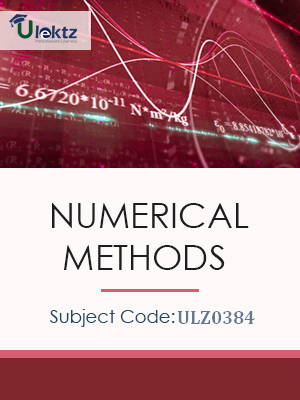•My WalletMy Order
•My Profile
•My Connections
•My Books
•My Videos
•My Tests
•My Calender
•My Messages
•My Shopping Cart
•My Orders
•Account Settings
•Help

# Book Details# Numerical Methods

 Course Code : ULZ0384 Author : uLektz University : General for All University Regulation : 2017 Categories : Civil Format :ePUB3 (DRM Protected) Type : eBook

FREE

Description :Numerical Methods of ULZ0384 covers the latest syllabus prescribed by General for All University for regulation 2017. Author: uLektz, Published by uLektz Learning Solutions Private Limited.

Note : No printed book. Only ebook. Access eBook using uLektz apps for Android, iOS and Windows Desktop PC.

##### Topics
###### UNIT I SOLUTION OF EQUATIONS AND EIGENVALUE PROBLEMS

1.1 Solution of algebraic and transcendental equations

1.2 Fixed point iteration method

1.3 Newton Raphson method

1.4 Solution of linear system of equations

1.5 Gauss elimination method

1.6 Pivoting

1.7 Gauss Jordan method

1.8 Iterative methods of Gauss Jacobi and Gauss Seidel

1.9 Matrix Inversion by Gauss Jordan method

1.10 Eigen values of a matrix by Power method

###### UNIT II INTERPOLATION AND APPROXIMATION

2.1 Interpolation with unequal intervals - Lagrange's interpolation

2.2 Newton’s divided difference interpolation

2.3 Cubic Splines

2.4 Interpolation with equal intervals - Newton’s forward and backward difference formula

###### UNIT III NUMERICAL DIFFERENTIATION AND INTEGRATION

3.1 Approximation of derivatives using interpolation polynomials

3.2 Numerical integration using Trapezoidal, Simpson’s 1/3 rule – Romberg’s method

3.3 Two point and three point Gaussian quadrature formula

3.4 Evaluation of double integrals by Trapezoidal and Simpson’s 1/3 rules

###### UNIT IV INITIAL VALUE PROBLEMS FOR ORDINARY DIFFERENTIAL EQUATIONS

4.1 Single Step methods

4.2 Taylor’s series method

4.3 Euler’s method - Modified Euler’s method

4.4 Fourth order Runge-Kutta method for solving first order equations

4.5 Multi step methods - Milne’s and Adams-Bash forth predictor corrector methods for solving first order equations

###### UNIT V BOUNDARY VALUE PROBLEMS IN ORDINARY AND PARTIAL DIFFERENTIAL EQUATIONS

5.1 Finite difference methods for solving two-point linear boundary value problems

5.2 Finite difference techniques for the solution of two dimensional Laplace’s and Poisson’s equations on rectangular domain

5.3 One dimensional heat flow equation by explicit and implicit (Crank Nicholson) methods

5.4 One dimensional wave equation by explicit method

### Related Books